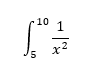## Definite Integrals

Calculate the definite integral shown.Hint
$$\int x^n \:dx=\frac{x^{n+1}}{n+1}$$$Hint 2 After solving for the indefinite integral, plug in 10 as the upper integral boundary and 5 as the lower integral limit. This is a two step calculus problem. First, let’s applying the power rule to solve the integral: $$\int \frac{1}{x^2}dx=\frac{x^{n+1}}{n+1}\:with\:n=-2$$$
$$\int (x^{-2})dx=-\frac{1}{x}+C$$$Next, solve using the upper integral boundary of 10, and lower integral limit of 5: $$(\frac{-1}{x})-(\frac{-1}{x})\rightarrow -\frac{1}{10}+\frac{1}{5}=-0.1+0.2=0.1$$$
0.1